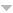# Publications

Found 42 results
[ Author] Title Type Year
Filters: First Letter Of Last Name is R and Author is Rosales, J. C.  [Clear All Filters]
R
Constructing almost symmetric numerical semigroups from irreducible numerical semigroups. Comm. Algebra. 2014;42:1362-1367.Edit
Every numerical semigroup is one half of infinitely many symmetric numerical semigroups. Comm. Algebra. 2008;36:2910-2916.Edit
The set of solutions of a proportionally modular Diophantine inequality. J. Number Theory. 2008;128:453-467.Edit
Numerical semigroups having a Toms decomposition. Canad. Math. Bull.. 2008;51:134-139.Edit
Every numerical semigroup is one half of a symmetric numerical semigroup. Proc. Amer. Math. Soc.. 2008;136:475-477 (electronic).Edit
Numerical semigroups with maximal embedding dimension [\refcno 2056070]. In: Focus on commutative rings research. Nova Sci. Publ., New York; 2006. 4. p. 47-53p. Edit
Modular Diophantine inequalities and numerical semigroups. Pacific J. Math.. 2005;218:379-398.Edit
Pseudo-symmetric numerical semigroups with three generators. J. Algebra. 2005;291:46-54.Edit
Numerical semigroups with a monotonic Apéry set. Czechoslovak Math. J.. 2005;55(130):755-772.Edit
$k$-factorized elements in telescopic numerical semigroups. In: Arithmetical properties of commutative rings and monoids. Vol 241. Chapman & Hall/CRC, Boca Raton, FL; 2005. 2. p. 260-271p. (Lect. Notes Pure Appl. Math.; vol 241).Edit
Numerical semigroups. Vol 20 Springer, New York 2009.Edit
Numerical semigroups with embedding dimension three. Arch. Math. (Basel). 2004;83:488-496.Edit
Fundamental gaps in numerical semigroups with respect to their multiplicity. Acta Math. Sin. (Engl. Ser.). 2004;20:629-646.Edit
Saturated numerical semigroups. Houston J. Math.. 2004;30:321-330 (electronic).Edit
Arf numerical semigroups. J. Algebra. 2004;276:3-12.Edit
Fundamental gaps in numerical semigroups. J. Pure Appl. Algebra. 2004;189:301-313.Edit
Atomic commutative monoids and their elasticity. Semigroup Forum. 2004;68:64-86.Edit
Numerical semigroups with maximal embedding dimension. Int. J. Commut. Rings. 2003;2:47-53.Edit
Proportionally modular Diophantine inequalities. J. Number Theory. 2003;103:281-294.Edit
The oversemigroups of a numerical semigroup. Semigroup Forum. 2003;67:145-158.Edit
Ideals of finitely generated commutative monoids. Semigroup Forum. 2003;66:305-322.Edit
Presentations of finitely generated submonoids of finitely generated commutative monoids. Internat. J. Algebra Comput.. 2002;12:659-670.Edit
Systems of inequalities and numerical semigroups. J. London Math. Soc. (2). 2002;65:611-623.Edit